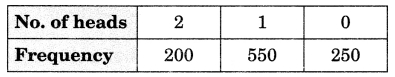CBSE Class 9 Probability MCQ New

## CBSE Class 9 Probability MCQ By Clarify Knowledge

CBSE Class 9 Probability MCQ New Pattern 2022

## CBSE Class 9 Probability MCQ Table

Question 1.
Which of the following cannot be the empirical probability of an event?
(a) 23
(b) 32
(c) 0

Question 2.
In a survey of 364 children aged 19-36 months, it was found that 91 liked to eat potato chips. If a child is selected at random, the probability that he/she does not like to eat potato chips is:
(a) 0.25
(b) 0.50
(c) 0.75

Question 3.
In a sample study of 640 people, it was found that 512 people have a high school certificate. If a person is selected at random, the probability that the person has a high school certificate is:
(a) 0.5
(b) 0.6
(c) 0.7

Question 4.
The probability that a number selected at random from the numbers 1, 2, 3, …, 15 is a multiple of 4 is
(a) 415
(b) 215
(c) 15

Question 5.
When a die is thrown, the probability of getting an odd number less than 4 is
(a) 16
(b) 13
(c) 12

Question 6.
A bag contains 16 cards bearing number 1, 2, 3 …., 16 respectively. One card is drawn at random. What is the probability that a number is divisible by 3?
(a) 316
(b) 516
(c) 1116

Question 7.
In a cricket match a batsman hits a boundary 4 times out of the 32 balls he plays. In a given ball, what is the probability that he does not hit the ball to the boundary?
(a) 78
(b) 18
(c) 17

Question 8.
The sum of the probabilities of all events of a trial is
(a) 1
(b) greater than 1
(c) less than 1

Question 9.
A die is thrown 300 times and odd numbers are obtained 153 times. Then the probability of getting an even number is
(a) 153300
(b) 147300
(c) 174300